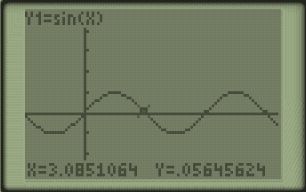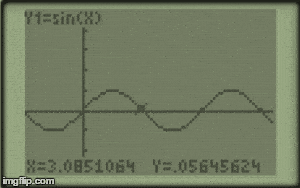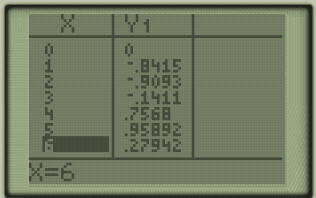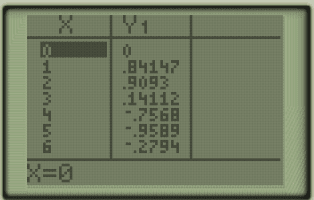# Trig functions: Amplitude

Our basic y=sin (x) function looks like this, with the y axis coming in increments of 1 and the x axis coming in increments of π .y = sin (x)

Now, what happens if the coefficient of sin(x) is changed from 1 (assumed  but not written) to -1?

Here’s a gif of it…negative to positive sin(x)

all the y-coordinates are now negative where they were previously positive in y=sin(x).y=-sin(x)

And for y=sin(x) all y coordinates which were negative are now positive.y=sin(x)

If we looks at a function such as y=3sin(x) we can further see the effect this coeffient A has on the function (y=Asin(x)).

y=3sinx

The period of 2π is still the same as y=sin(x).y=3sin(x)

Yet the amplitude (distance from midline to high or low point on graph is now 3.

In our next post we’ll look at determining the period of basic trig functions.